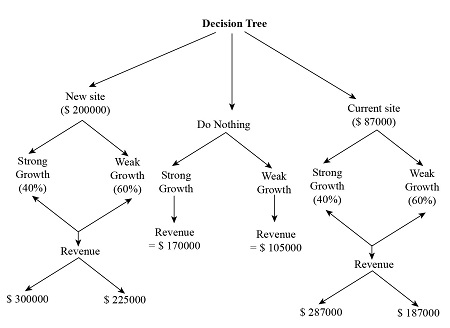# The owner of a cellphone store is evaluating three options-expand at current site, expand to a...

## Question:

The owner of a cellphone store is evaluating three options-expand at current site, expand to a new site, and do nothing. The decision process includes the following assumptions and conditions:

1. Strong growth has a 40% probability

2. If they expand to a new site:

- cost =$200,000 Revenue= Strong growth=$300,000 Weak growth:$225,000 3. If expand at current site Cost=$87,000

Revenue= Strong growth=$287,000 Weak growth=$187,000

4. If do nothing:

Revenue=Strong growth=$170,000 Weak growth=$105,000

A. Construct a decision tree

B. Solve the tree

C. What should this firm decide to do based on a decision tree evaluation?

D. Provide critical thinking on the decision tree evaluation

## Expected Payoff

Expected Payoff is the value of money which the decision maker or investor expect to receive considering the best and worst scenario both. It is calculated by minimizing the probabilistic value of loss from the probabilistic value of gain.

(A)

The decision tree including all the three alternative with their respected value of cost and revenue:(B)

{eq}Expected Payoff = [(Revenue - Cost) \times P of strong Growth] - [(Revenue - Cost) \times P of Weak Growth] {/eq}

Option: New Site

{eq}\begin{align*} {\rm{Expected}}\,{\rm{Payoff}} &= [(\$300,000 - \$ 200,000) \times 0.4] - [(\$225,000 - \$ 200,000) \times 0.6]\\ &= (\$100000 \times 0.4) - (\$ 25000 \times 0.6)\\ &= \$40000 - \$ 15000\\ &= \25000 \end{align*} {/eq} Option: Current Site {eq}\begin{align*} {\rm{Expected}}\,{\rm{Payoff}} &= [(\ 287,000 - \$87,000) \times 0.4] - [(\$ 187,000 - \$87,000) \times 0.6]\\ &= (\$ 200000 \times 0.4) - (\$100000 \times 0.6)\\ &= \$ 80000 - \$60000\\ &= \$ 20000 \end{align*} {/eq}

Option: Do Nothing

{eq}\begin{align*} {\rm{Expected}}\,{\rm{Payoff}} &= (\$170,000 \times 0.4) - (\$ 105,000 \times 0.6)\\ &= \$68000 - \$ 63000\\ &= \\$ 5000 \end{align*} {/eq}

(C)

The expected payoff value is highest in the option of expansion at new site. Therefore, company should select the option of expansion at New Site.

(D)

The Critical Thinking approach used in this way:

• All the alternatives are analysed by the probability of strong growth and weak growth.

• The profit under each alternative is determined.

• The expected payoff is calculated under each alternative to evaluate the options.

• The decision is taken on the basis of which option is giving highest expected value.